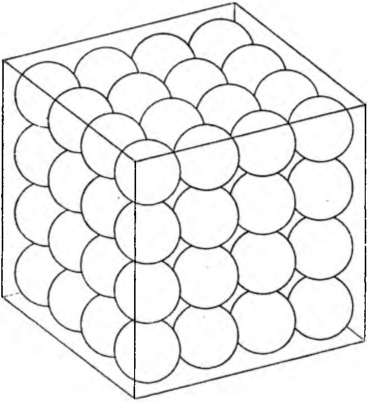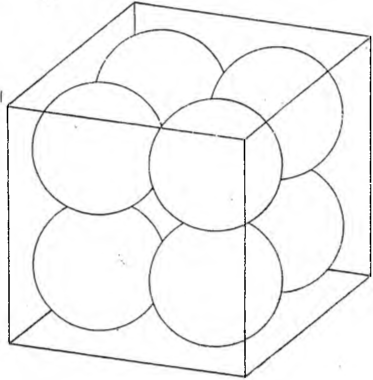# Chest with ballsThe factory sent a cubic crate with steel balls. The empty chest weighed 2 kg, and the gross weight of the box with the balls was 18 kg. They were in the chest 64 the same balls arranged in four layers: at the bottom of the box lay a layer of four rows of four balls, on it the second, same layer, above the third, finally the fourth layer.

There was a second chest of the same type 1000 balls arranged in ten layers, and in each layer there were ten rows of ten marbles in a row. How much did this box weigh gross?

The balls in the first box weighed net 16 kg, and because there were balls 64, so each ball weighed 0,25 kg.

Let's think about it, how much would one large ball weigh, that would touch all six sides of the box.

Such a sphere would have a diameter 4 times greater than either of 64 balls sent in the first crate. The weight of this ball would be 4 • 4 • 4 = 64 times greater than the weight of each of 64 balls sent in the first crate, so one large ball would weigh just that, co 64 balls shipped in the first crate, or 16 kg.If we now take balls with a diameter of 2 times smaller than the diameter of a large sphere, it will fit in the chest 2 • 2 • 2 = 8 kul, and the total burden will be this, what a great ball has!

If we took balls with a diameter of 3 times smaller than the diameter of a large sphere, it would be bullets 3 • 3 • 3 = 27, and the total burden would remain the same.

Now we can answer the question, how much weighed a box with a thousand balls:

16 kg net weight, a 18 kg gross weight.

Finally, calculate, how much would such a box weigh, if it was filled with steel shots arranged in equal layers!